# [Processing × Java] How to use loop 2 --- Nested structure, coordinate conversion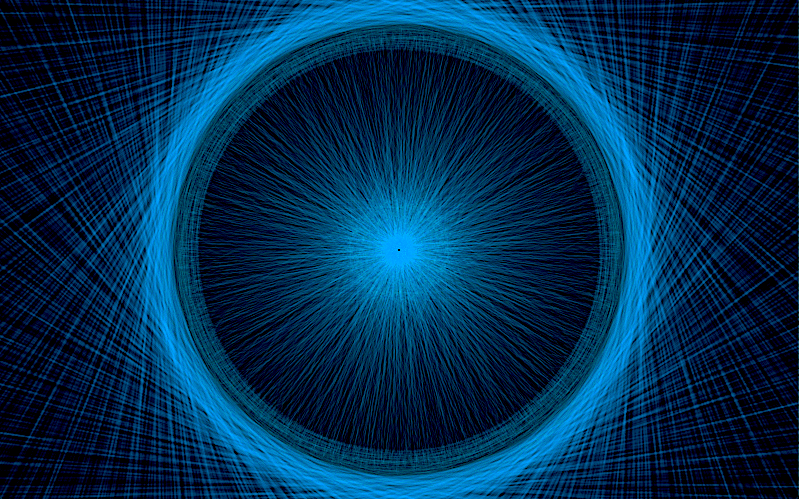# 0.for loop

## 0-0. ** for loop and coordinate transformation **

In Processing, you can customize "** reference point when drawing a figure " and " coordinates themselves **" to your convenience. At that time, the for loop is often used together. Below, I will write about coordinate transformation using a for loop.

### ◯ Program to move the reference point of "figure"

#### `for00.java`

``````
size(500,500);
background(255);
//Make it possible to draw a figure based on the center of the rectangle.
rectMode(CENTER);

//Repeat 5 times(i = 0,1,2,3,4)
for(int i = 0;i < 5;i++){
//Define an int type variable s that represents the size of the sides of the quadrangle.
int s = 400 -i * 80;

noFill();
stroke(115,237,201);
//Determine the thickness of the border
strokeWeight(3);
//The center is(width/2,height/2)Then draw a rectangle with s sides.
rect(width/2,height/2,s,s);
}
``````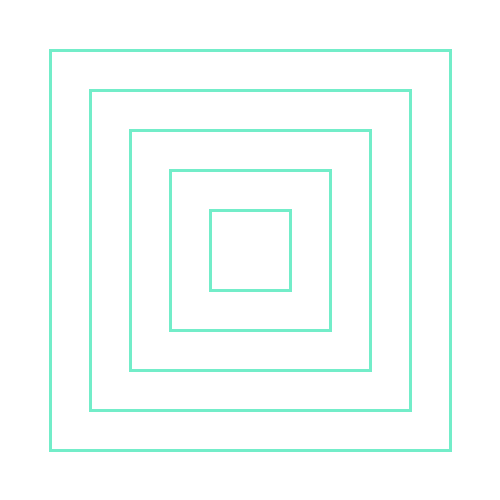Point : rectMode(CENTER) You can use it to draw shapes relative to the center of the rectangle **. (The default reference point is the upper left corner of the rectangle)

### ◯ A program that shifts the reference point of "coordinates"

The following program uses translate () to shift the center of the coordinates to (width / 2, height / 2). In other words, the coordinates of the center of the screen (Processing window) are now (0,0).

#### `for01.java`

``````
size(500,500);
background(255);
//Coordinate reference point(origin)The center of the screen(width/2,height/2)Shift
translate(width/2,height/2);

//Repeat 3 times
for(int i = 0;i < 3;i++){
//TWO_PI/6 =60 degrees, rotate every loop
rotate(TWO_PI/6);
noFill();
stroke(12,232,178);
//The center of the screen(0,0)So...
ellipse(0,0,150,375);
}
``````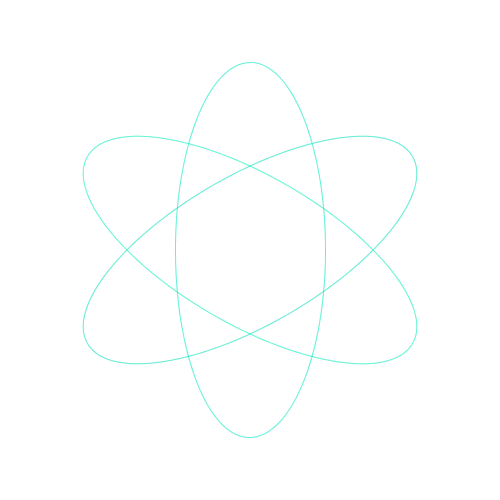Point : translate(); A function for shifting the reference point of coordinates. This time, the reference point was moved to the center of the screen.

Point : rotate(); Rotate around the reference point of the coordinates. This time, the reference point is in the center of the screen, so I rotated around it. Arguments are specified using ** PI ** and ** TWO_PI **.

Point : TWO_PI 2π = 360 degrees The angle is expressed by the length of the arc in a form called radian. The circumference of the unit circle with radius 1 is 2 * π = 2π. The arc 2π of the unit circle is the angle of one circle = 360 degrees.

## 0-1. ** for loop and nesting structure **

### ◯ Double nesting program

#### `for02.java`

``````
size(510,510);
background(255);
stroke(12,232,178);

//Repeat horizontal movement 5 times(i = 10,110,210,310,410)
for(int i = 10;i < width;i += 100){
//Repeat vertical movement 5 times(j = 10,110,210,310,410)
for(int j = 10;j < height;j += 100){
fill(12,232,178,255-i * 0.5);
rect(i,j,80,80);
}
}
``````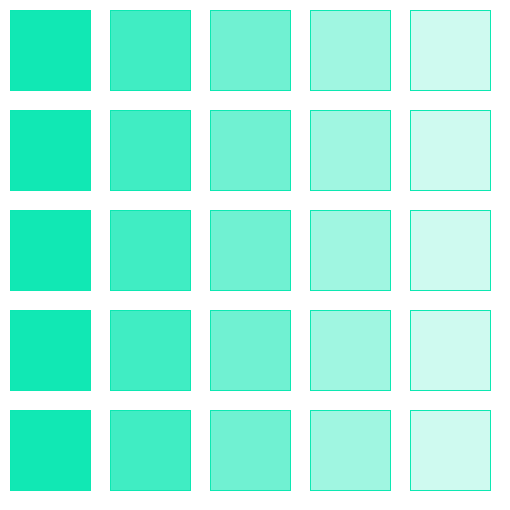** Point **: Program execution order

In the case of this program, it will be executed in order from the leftmost column (from the top).

In other words ** When i = 10 ** ・ J = 10: Execute ・ J = 110: Execute ・ J = 210: Execute ・ J = 310: Execute ・ J = 410: Execute ** When i = 110 ** ・ J = 10: Execute ・ J = 110: Execute ・ J = 210: Execute ・ J = 310: Execute ・ J = 410: Execute ** When i = 210 ** 　　　　:

### ◯ Double nesting program (animation)

#### `draw.java`

``````
//I want to change the color, so prepare the variable c
int c = 0;

void setup(){
size(510,510);
}

//The variable c is gradually shifted.
void draw(){
//Repeat horizontal movement 500 times(i = 0,1,2...508,509)
for(int i = 0;i < width;i ++){
//Repeat vertical movement 500 times(j = 0,1,2...508,509)
for(int j = 0;j < height;j ++){
stroke(c,255-j/2,i/2);
//Draw a 1-pixel dot
point(i,j);
}
}
c += 10;
//Export colors
println(c);
//If the value of variable c exceeds 255
if(c > 255){
//Set c to 0
c = 0;
}
}
//Runs only once when the mouse is pressed
void mousePressed(){
//Save the window when the mouse is pressed in png format
save("draw"+ c +".png ");
//Output that the image has been saved
println("picture is saved.");
}
``````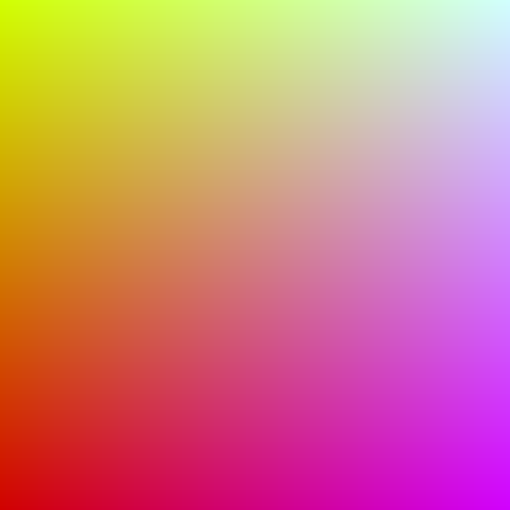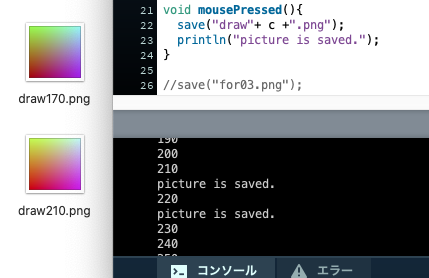Point :save() Images can be saved. Write the name and save format in "", such as save ("~ .png ") ;. You can check the saved image by selecting [Open Sketch Folder] from [Sketch] on the menu bar.

### ◯ Mie nesting program

#### `for03.java`

``````
size(510,510);
background(255);
noFill();
stroke(12,232,178);
//Be able to draw based on the center of the rectangle
rectMode(CENTER);

//Repeat 5 times(i = 55,155,255,355,455)
for(int i = 55;i < width;i += 100){
//Repeat 5 times(j = 55,155,255,355,455)
for(int j = 55;j < height;j += 100){
//Repeat 4 times(s = 10,30,50,70)This completes one square section.
for(int s = 10;s < 90;s += 20){
//Coordinate(i,j)Then draw a square with a side size of s
rect(i,j,s,s);
}
}
}
``````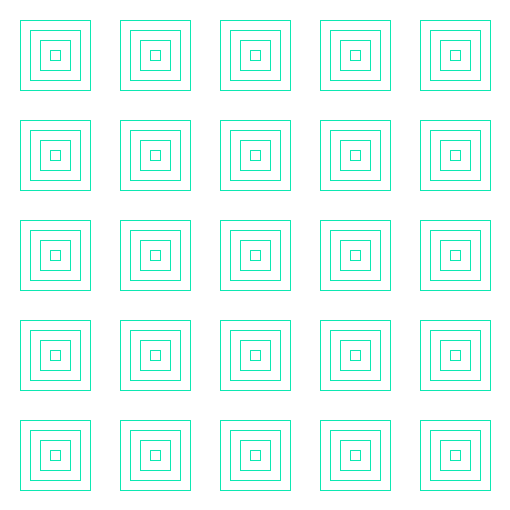Point : ** Matryoshka-like ** chunks of 4 squares of different sizes **

``````for(int s = 10;s < 90;s += 20){
rect(,,s,s);
}
``````

Part of.

Arrange these chunks (same as in the double loop).

# Finally

Thank you for reading. We appreciate your opinions and suggestions in order to make the article even better.